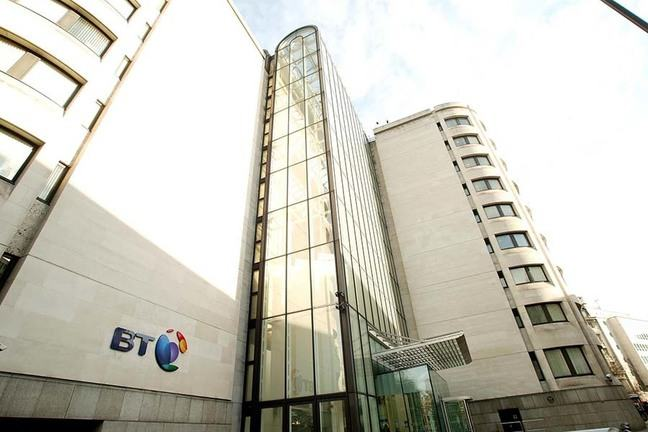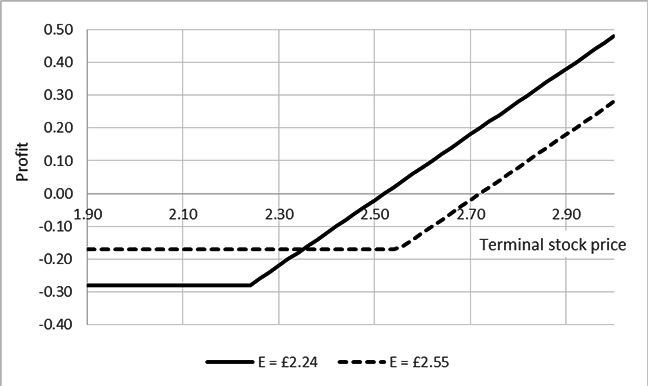3.16

## SOAS University of LondonBT Centre, Newgate Street, London

# Options profits: an application

In this article we will consider a call option on the stock of BT Group, the UK telecommunications company. We will use the Black-Scholes model to price the option, and consider the potential profits from purchasing the call option.

Suppose the price of BT stock is currently £2.24 per share, and you think the share price will increase over the next 12 months. What strategies might you follow to take advantage of your expectation?

You could purchase BT shares for £2.24 per share, and hold them for a year or more. But what if your expectation is incorrect, and the share price falls in that time?

Alternatively, you could purchase a call option on BT shares. You might choose the exercise price to be £2.24, and obtain the right to purchase BT shares at £2.24. After 12 months, if the share price is more than £2.24 you would exercise the option, purchase the shares for £2.24, and sell the shares at the higher price. If the share price is less than £2.24 after 12 months, you would leave the option unexercised.

To determine the price of this option we can use the Black-Scholes model. The inputs are the stock price at the time the option is purchased; exercise price; time to maturity; volatility of returns on BT stock; and the risk-free rate of return.

In this case the starting stock price is £2.24. We have chosen an exercise price also equal to £2.24. Time to maturity is one year. For a risk-free rate of return we can use LIBOR (the London Interbank Offered Rate) or the Bank of England’s SONIA (sterling overnight index average) lending rate. We have chosen a value of 0.70%.

You examined daily returns on BT Group stock in the additional example in step 3.13. The standard deviation of BT daily returns is 1.9%. The corresponding estimate of the annual standard deviation is 31%, and we use this value for the annual volatility. The relation between daily standard deviation and annual standard deviation is

$1.9$% $\times$ $\sqrt{252}$ = $31$%

(we have assumed 252 trading days a year).

Using these inputs, the price of a call option is £0.28.

In the figure below, the solid line marked E = £2.24 shows the profit from a long position in this option, for a range of values of the terminal stock price when the option matures. The profit is the payoff from the option minus the option premium.

For terminal stock prices below £2.24 the option will not be exercised. The payoff is zero and the loss is the premium paid, £0.28. For prices above £2.24, the option will be exercised and will generate a positive payoff. However, for terminal stock prices between £2.24 and £2.52 the payoff is less than the premium, and the option makes a loss (although the loss is less than £0.28). For terminal stock prices greater than £2.52 the option would make a profit.Profit pattern, long call on BT stock

Suppose you chose a higher exercise price, say £2.55. What is the effect on the option premium and the profit pattern?

For an exercise price of £2.55, the option premium is £0.17 using the Black-Scholes model. Can you explain why the premium for the call option is lower if the exercise price is higher? There is less chance the terminal stock price will be above the exercise price at maturity, and less chance you will exercise the option.

The dashed line illustrates the profit pattern for a call option with exercise price £2.55. The option would be exercised if the terminal stock price is above £2.55, and will generate a profit if the terminal stock price is higher than £2.72.

This article provides an example of option pricing, and the profits from holding call options. What are your thoughts on the inputs we have used to price the option? And on the strategy we have described?